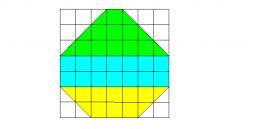# Isosceles trapezoid

Calculate the area of an isosceles trapezoid whose bases are in the ratio of 4:3; leg b = 13 cm and height = 12 cm.

Correct result:

S =  420 cm2

#### Solution:

$S = (a+c)h/2 \ \\ \ \\ a_1^2 = b^2 - h^2 \ \\ a_1 = \sqrt{b^2 - h^2} = \sqrt{ 13^2 - 12^2} = 5 \ cm \ \\ a = c+2a_1 \ \\ a/c = 4/3 \ \\ (c+2a_1)c = 4/3 \ \\ 3(c+2a_1) = 4c \ \\ 6a_1 = c \ \\ c = 6a_1 = 6 \cdot 5 = 30 \ cm \ \\ a = c + 2a_1= 30 + 2\cdot 5 = 40 \ cm \ \\ \ \\ S = (a+c)h/2 = (40 + 30) \cdot 12 /2 = 420 \ \text{cm}^2 \ \\$Our examples were largely sent or created by pupils and students themselves. Therefore, we would be pleased if you could send us any errors you found, spelling mistakes, or rephasing the example. Thank you!

Please write to us with your comment on the math problem or ask something. Thank you for helping each other - students, teachers, parents, and problem authors.Tips to related online calculators
Pythagorean theorem is the base for the right triangle calculator.

#### You need to know the following knowledge to solve this word math problem:

We encourage you to watch this tutorial video on this math problem:

## Next similar math problems:

• EmbankmentPerpendicular cross-section of the embankment around the lake has the shape of an isosceles trapezoid. Calculate the perpendicular cross-section, where bank is 4 m high the upper width is 7 m and the legs are 10 m long.
• Isosceles trapeziumCalculate the area of an isosceles trapezium ABCD if a = 10cm, b = 5cm, c = 4cm.
• Parcelparcel has a rectangular shape of a trapezoid with bases 12 m and 10 m and a height 8 m. On parcel was built object with a footprint an isosceles triangle shape with side 4 m and height three-quarters of a meter. What is the area of unbuild parcel?
• ISO trapezoid v2bases of Isosceles trapezoid measured 16 cm and 4 cm and its perimeter is 47 cm. What is the are of a trapezoid?
• Isosceles trapezoidWhat is the height of an isosceles trapezoid, the base of which has a length of 11 cm and 8 cm and whose legs measure 2.5 cm?
• Rectangular trapezoidCalculate the content of a rectangular trapezoid with a right angle at the point A and if |AC| = 4 cm, |BC| = 3 cm and the diagonal AC is perpendicular to the side BC.
• EstateEstate shaped rectangular trapezoid has bases long 34 m , 63 m and perpendicular arm 37 m. Calculate how long is its fence.
• Equilateral triangleThe equilateral triangle has a 23 cm long side. Calculate its content area.
• The right triangleThe right triangle ABC has a leg a = 36 cm and an area S = 540 cm2. Calculate the length of the leg b and the median t2 to side b.
• StairwayStairway has 20 steps. Each step has a length of 22 cm and a height of 15 cm. Calculate the length of the handrail of staircases if on the top and bottom exceeds 10 cm.
• Median in right triangleIn the rectangular triangle ABC has known the length of the legs a = 15cm and b = 36cm. Calculate the length of the median to side c (to hypotenuse).
• Cone 15The radius of the base of a right circular cone is 14 inches and it's height 18 inches. What is the slant height?The double ladder shoulders should be 3 meters long. What height will the upper top of the ladder reach if the lower ends are 1.8 meters apart?A tree at a height of 3 meters broke in the windbreak. Its peak fell 4.5 m from the tree. How tall was the tree?The double ladder is 8.5m long. It is built so that its lower ends are 3.5 meters apart. How high does the upper end of the ladder reach?It is true that the middle traverse bisects the triangle?If a, b and c are two sides of a triangle ABC, a right angle in A, find the value on each missing side. If b=10, c=6